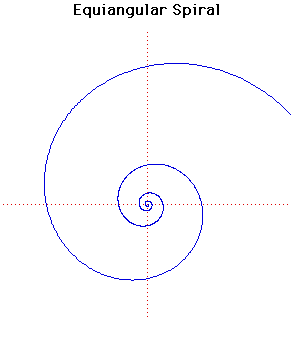# Equiangular SpiralPolar equation:
r = a exp(θ cot b)

Click below to see one of the Associated curves.

Click THIS LINK to experiment interactively with this curve and its associated curves.

The equiangular spiral was invented by Descartes in 1638. Torricelli worked on it independently and found the length of the curve.

If P is any point on the spiral then the length of the spiral from P to the origin is finite. In fact, from the point P which is at distance d from the origin measured along a radius vector, the distance from P to the pole is d sec b. Jacob Bernoulli in 1692 called it spira mirabilis and it is carved on his tomb in Basel.

It occurs naturally in many places like sea-shells where the growth of an organism is proportional to the size of the organism. In his book Growth and Form, D'Arcy Thompson devoted a whole chapter to this curve and describes its occurence in Nature as the result of coiling a cone upon itself, contrasting it with the Spiral of Archimedes which is formed by coiling a cylinder as a sailor coils a rope upon the deck.

You can see this
shell of the many-chambered Nautilus or the shell with the curve super-imposed.

The spiral make a constant angle b with any radius vector. Of course in the special case where b = π/2 one obtains a circle. For the curve displayed above b = /16. Therefore the length of the curve from a point at distance d from the origin along a radius vector is about 5.126 d.

Any radius from the origin meets the spiral at distances which are in geometric progression.

The pedal of an equiangular spiral, when the pedal point is the pole, is an identical equiangular spiral.

The evolute and the involute of an equiangular spiral is an identical equiangular spiral. This was shown by Johann Bernoulli.

The caustic of the equiangular spiral, where the pole is taken as the radiant, is an equal equiangular spiral.

Other Web site:

JOC/EFR/BS January 1997